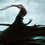# A Flag Problem!!

On Some Planet,there are $2^n$ Countries ($n \geq 4$ ).

Each Country Has a Flag $n$ units wide and one unit high composed of $n$ Fields of size $1 \times 1$,each field being either yellow or blue.No two countries have the same flag.

We say that a set of $n$ flags is diverse if these flags can be Arranged in $n \times n$ square so that all $n$ fields on its Main Diagonal will have the same colour.

Determine the Smallest Possible integer $m$ such that among any $m$ distinct flags,there exist $n$ Flags forming a Diverse Set.

I Need A bit of help In Understanding the Last Part of the Problem.Note by Vraj Mehta
6 years, 3 months ago

This discussion board is a place to discuss our Daily Challenges and the math and science related to those challenges. Explanations are more than just a solution — they should explain the steps and thinking strategies that you used to obtain the solution. Comments should further the discussion of math and science.

When posting on Brilliant:

• Use the emojis to react to an explanation, whether you're congratulating a job well done , or just really confused .
• Ask specific questions about the challenge or the steps in somebody's explanation. Well-posed questions can add a lot to the discussion, but posting "I don't understand!" doesn't help anyone.
• Try to contribute something new to the discussion, whether it is an extension, generalization or other idea related to the challenge.
• Stay on topic — we're all here to learn more about math and science, not to hear about your favorite get-rich-quick scheme or current world events.

MarkdownAppears as
*italics* or _italics_ italics
**bold** or __bold__ bold
- bulleted- list
• bulleted
• list
1. numbered2. list
1. numbered
2. list
Note: you must add a full line of space before and after lists for them to show up correctly
paragraph 1paragraph 2

paragraph 1

paragraph 2

[example link](https://brilliant.org)example link
> This is a quote
This is a quote
    # I indented these lines
# 4 spaces, and now they show
# up as a code block.

print "hello world"
# I indented these lines
# 4 spaces, and now they show
# up as a code block.

print "hello world"
MathAppears as
Remember to wrap math in $$ ... $$ or $ ... $ to ensure proper formatting.
2 \times 3 $2 \times 3$
2^{34} $2^{34}$
a_{i-1} $a_{i-1}$
\frac{2}{3} $\frac{2}{3}$
\sqrt{2} $\sqrt{2}$
\sum_{i=1}^3 $\sum_{i=1}^3$
\sin \theta $\sin \theta$
\boxed{123} $\boxed{123}$

## Comments

Sort by:

Top Newest

Any Ideas?

- 6 years, 3 months ago

Log in to reply

The last part of the question is asking the value of the smallest possible value of $m$ such that if you take any $m$ flags from the $2^n$ flags, you can always find $n$ flags from those $m$ flags such that those $n$ flags form a "diverse" set.

$m \geq n$ is implicit, otherwise we wouldn't be able to take $n$ flags from the $m$ flags.

Is this question from a Maths Magazine for JEE? (Can't remember the name).

- 6 years, 3 months ago

Log in to reply

Yes,Its from that Magazine,"Mathematics Today"..

Thanks,I got the meaning now,Perphaps now everyone can solve it.

- 6 years, 3 months ago

Log in to reply

They should also clarify if "main diagonal" refers to just 1 diagonal, or both. As it turns out, it's just 1 diagonal, ie. squares of the form $(i,i)$.

This is IMO Shortlist 2010 C2, which I believe is the primary source.

This is essentially a construction problem and there are 2 main parts to it.
We have have to guess what $M$ is going to be, and explain why $M - 1$ doesn't work. That's the easy part.

We then have to show that given $M$ flags, we can always find $N$ that satisfy the condition. There is a solution using the "backward induction" approach, but the base case requires quite a bit of work.

There is another solution using the Hall Marriage Theorem, but that would require more understanding.

Staff - 6 years, 3 months ago

Log in to reply

×

Problem Loading...

Note Loading...

Set Loading...